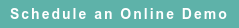# RoyaltyStat Blog

### Posts by Topic

Determining an arm’s length profit indicator (aka profit ratio) requires two equations, and not one equation, as prescribed in financial statement analysis textbooks. E.g., Bernstein (1993), Drake & Fabozzi (2012). An accounting critique of univariate profit ratios is found in Whittington (1986).

The accounting equation is true by definition and is not subject to controversy. The behavioral equation has profits (dependent variable) as a function of specific X-factors. In reduced form, the behavioral equation is subject to empirical testing. We illustrate the two-equations profit determination system using return on assets (ROA):

(1) S(t) = C(t) + d K(t – 1) + P(t)

where S(t) is net sales of a selected enterprise in period t = 1 to T, C(t) is total cost consisting of COGS plus XSGA during the same period, K(t – 1) is the sum of PPENT and INTANO at the beginning of the period, and P(t) is OIADP (operating profit after depreciation and amortization). These variables are defined by using acronyms from Standard & Poor’s Compustat® database of publicly traded company financials. Operating assets = (PPENT + INTANO) because the depreciation rate includes the amortization rate. The stock variable K(t – 1) must be lagged one period because of the “law of motion of capital”. Klein (1983, pp. 9, 19).

A behavioral profit equation can be postulated such that annual profits are proportional to S(t) and K(t – 1), which is saying that the profit margin is adjusted for asset-intensity:

(2) P(t) = m S(t) + r K(t – 1) + U(t)

where U(t) is the uncertainty or random error.

Equation (2) may or may not include an intercept. Unlike the accounting equation (1), the postulated behavioral equation (2) is subject to statistical errors that may not satisfy standard assumptions, such as near-zero serial correlation among the residual errors.

Substitute equation (2) into (1) and obtain the reduced-form equation that is amenable to regression analysis:

(3) S(t) = C(t) + d K(t – 1) + m S(t) + r K(t – 1) + U(t)

(4) S(t) = β1 C(t) + β2 K(t – 1) + V(t)

The reduced-form equation (4) has two X-factors (independent variables), C(t) and K(t – 1).

The partial regression coefficients are the profit markup, β1 = 1 / (1 – m) > 1, and the composite ROA, β2 = [(d + r) / (1 – m)].

If ABS(m) < 1, which applies to the expected operating profit margin, we obtain the convergent series 1 / (1 – m) = 1 + m + m2 + m3 + …. In equation (4), S(t) is equivalent to a weighted sum of past total costs (or past investments). Lax & Terrell (2014, Theorem 1.13 (Geometric series), p. 29) or Thomas (1983, p. 564).

Because K(t – 1) is lagged one period (operating assets are measured at the beginning of the accounting year), the regression equation (4) must be run per individual company using its historical data.

If regression equation (4) produces β2 = 0, then model (4) reduces to a simple profit markup without asset intensity adjustment:

(5) S(t) = β1 C(t) + V(t)

Regression analysis based on comparable data using S(t), C(t), and K(t – 1) per company can determine the statistical validity of model (4) versus model (5). Unlike the parsimonious equation (5), we do not get consistent regression results using the expanded equation (4). A prevailing vice in transfer pricing is to assert model (4) without statistical testing. Another vice is to assert that β2 < 0, again without statistical testing.

#### References

Leopold Bernstein, Analysis of Financial Statements (4th edition), Irwin, 1993.

Pamela Drake & Frank Fabozzi, Analysis of Financial Statements (3rd edition), Wiley, 2012.

Lawrence Klein, Lectures in Econometrics, Elsevier, 1983.

Peter Lax & Maria Terrell, Calculus with Applications (2nd edition), Springer, 2014.

George Thomas, Calculus and Analytic Geometry (Classical edition), Addison-Wesley, 1983.

Geoffrey Whittington, “On the Use of the Accounting Rate of Return in Empirical Research,” in Richard Brief (editor), Estimating the Economic Rate of Return from Accounting Data, Routledge, 1986.

Published on Apr 28, 2023 12:00:27 PM

Ednaldo Silva (Ph.D.) is founder and managing director of RoyaltyStat. He helped draft the US transfer pricing regulations and developed the comparable profits method called TNNM by the OECD. He can be contacted at: esilva@royaltystat.com

RoyaltyStat provides premier online databases of royalty rates extracted from unredacted license agreements
and normalized company financials (income statement, balance sheet, cash flow). We provide high-quality data, built-in analytical tools, customer training and attentive technical support.